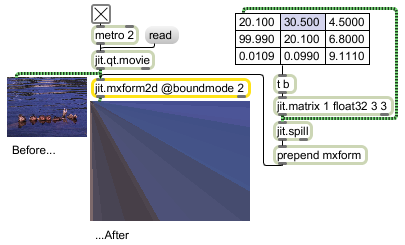# jit.mxform2d

Spatial transform using 3x3 matrix

## Description

The jit.mxform2d object performs a 2-dimensional matrix transform on an input matrix. It can be used to perform scaling, rotation, skewing, and perspective operations.

## Matrix Operator

matrix inputs:1, matrix outputs:1
 Name IOProc Planelink Typelink Dimlink Plane Dim Type out n/a 1 1 1 1 1 char long float32 float64

## Attributes

Name Type g/s Description
boundmode int Boundary handling mode (default = 3 (clip))
0 = ignore: Values that exceed the limits are ignored.
1 = clear: Values that exceed the limits are set to 0.
2 = wrap: Values that exceed the limits are wrapped around to the opposite limit with a modulo operation.
3 = clip: Values are limited not to exceed width or height.
4 = fold: Values that exceed the limits are folded back in the opposite direction.
interp int Interpolation flag (default = 0) If enabled, the object resamples the image and then interpolates back to the original size.
mxform float A list of nine variables ( a b u c d v tx ty w ) used in the matrix transform calculations (default 1. 0. 0. 0. 1. 0. 0. 0. 1.)
offset_x int The horizontal offset in pixels from the upper left corner for the displayed matrix (default = 0). Positive values shift right, negative values shift left.
offset_y int The vertical offset in pixels from the upper left corner for the displayed matrix (default = 0). Positive values shift down, negative values shift up.

## Examples